# 全同态加密图示导引

written by oneman233
2022-02-28

# 简介

HEANN https://github.com/kimandrik/HEAAN CC non commercial C++
NFLib https://github.com/quarkslab/NFLlib GPLv3 C++
FV-NFLib https://github.com/quarkslab/NFLlib GPLv3 C++
cuHE https://github.com/vernamlab/cuHE MIT CUDA C++
SEAL https://www.microsoft.com/en-us/research/project/simple-encrypted-arithmetic-library/ Microsoft research only C++
HELib https://github.com/shaih/HElib Apache 2.0 C++

• 一些数学介绍
• 加密解密工作流程
• 同态加法和乘法

# 一些数学介绍

$4x^2+2x+1$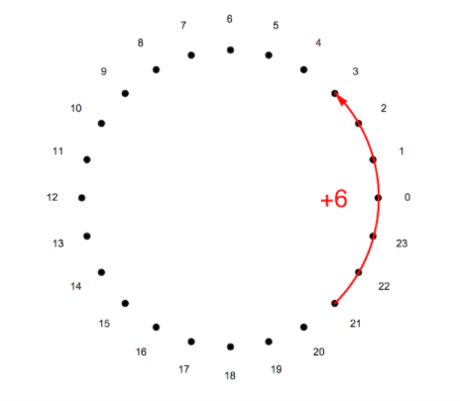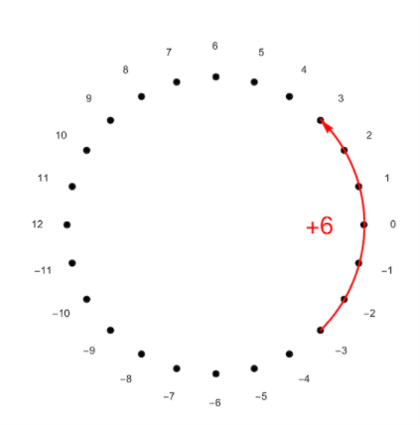\begin{aligned} & a_{15}x^{15}+a_{14}x^{14}+a_{13}x^{13}+a_{12}x^{12}+a_{11}x^{11}+a_{10}x^{10}+a_{9}x^{9}+ \\ & a_{8}x^{8}+a_{7}x^{7}+a_{6}x^{6}+a_{5}x^{5}+a_{4}x^{4}+a_{3}x^{3}+a_{2}x^{2}+a_{1}x+a_{0} \end{aligned}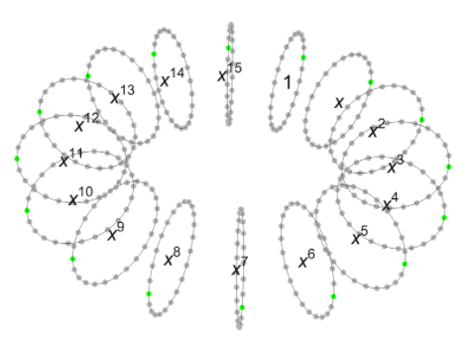FV策略包含很多多项式乘法，也就是把系数和次数分别相乘，例如当我们把 $2x^{14}$ 乘以 $x^4$ 时我们会得到 $2x^{18}$ ，别忘了我们还要把这个式子对我们的多项式模： $x^{16}+1$ 取模。如果只是简单地对 $x^{16}$ 取模，我们直接就能得到 $2x^2$ ，就像对整数取模的运算一样。但是这个加一运算引入了一个非常有意思的符号变化，这有助于我们进一步打乱乘法运算的结果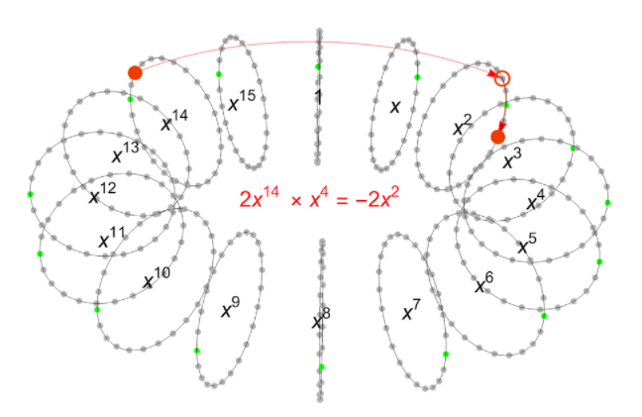# 加密解密工作流程

## 公钥和私钥

$s=x^{15}-x^{13}-x^{12}-x^{11}-x^9+x^8+x^6-x^4+x^2+x-1$

\begin{aligned} a= & 42x^{15}-256x^{14}-393x^{13}-229x^{12}+447x^{11}-369x^{10}-212x^9+ \\ & 107x^8+52x^7+70x^6−138x^5+322x^4+186x^3−282x^2−60x+84 \end{aligned}

\begin{aligned} e= & −3x^{15}+x^{14}+x^{13}+7x^{12}−6x^{11}−6x^{10}+ \\ & x^9+4x^8−x^6+3x^5−4x^4+4x^3+4x+1 \end{aligned}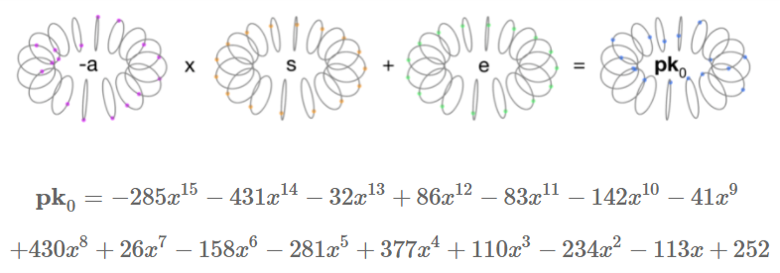## 加密

\begin{aligned} e1= & −5x^{15}−2x^{14}+3x^{13}−x^{12}−4x^{11}+3x^{10}+x^9+4x^8 \\ & +4x^7+5x^6−4x^5−3x^4−3x^3+2x^2−6x+4 \\ e2= & −7x^{15}+2x^{14}−4x^{13}+5x^{11}+2x^{10}−x^9+4x^8 \\ & −4x^7−3x^6+2x^5−2x^4+x^3−4x^2−2x+2 \\ u= & x^{14}+x^{13}+x^{12}−x^8−x^5−x^3+1 \end{aligned}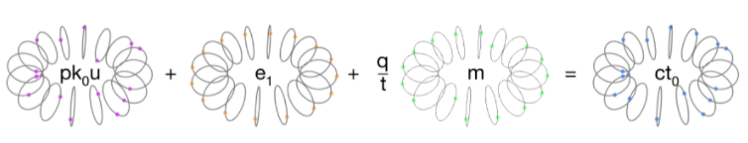\begin{aligned} CT_0= & 217x^{15}−53x^{14}+13x^{13}−249x^{12}−392x^{11}−238x^{10}+252x^9+115x^8 \\ & +5x^7+184x^6−201x^5−258x^4−247x^3+144x^2+23x+42 \end{aligned}

\begin{aligned} CT_1= & 25x^{15}+225x^{14}−12x^{13}+270x^{12}+350x^{11}−24x^{10}+56x^9−330x^8 \\ & +386x^7+225x^6−332x^5+68x^4−20x^3−26x^2−91x+380 \end{aligned}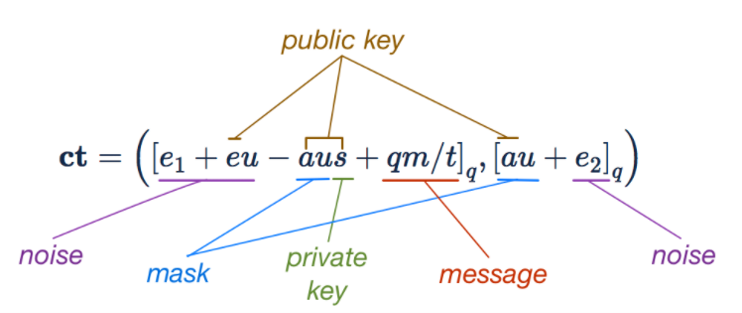## 解密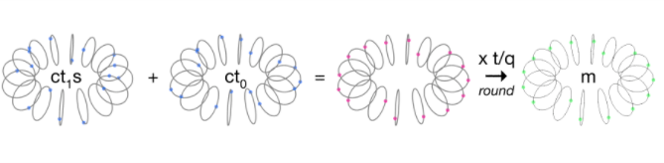\begin{aligned} CT_1*s+CT_0= & 13x^{15}−2x^{14}+17x^{13}+22x^{12}−32x^{11}−23x^{10}+19x^9−380x^8 \\ & +9x^7+10x^6−13x^5−3x^4−2x^3−12x^2+7x+393 \end{aligned}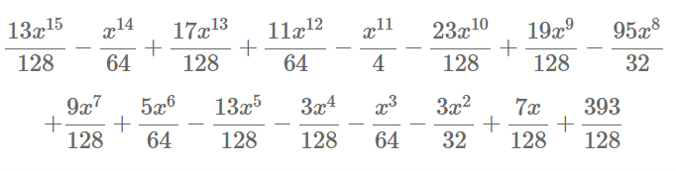$m=3-3x^8$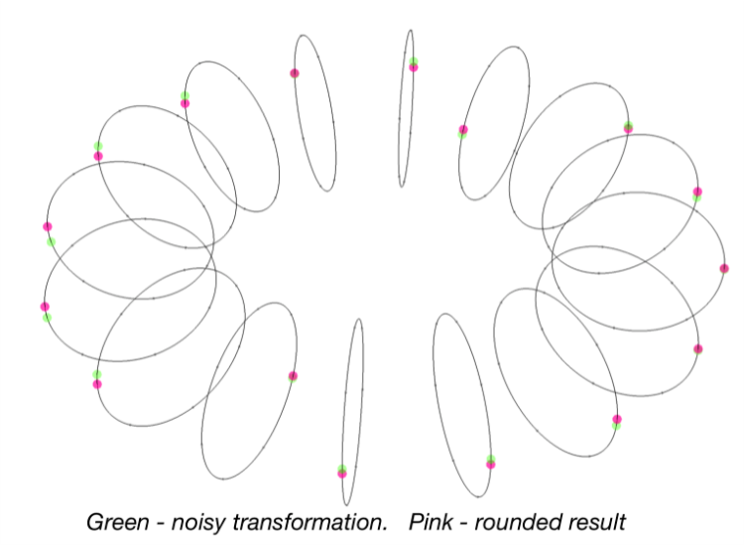$m'=[\lfloor \cfrac{t}{q}[CT_0+CT_1*s]_q \rceil]_t$

# 同态加法和乘法

## 同态加法

\begin{aligned} a=([PK_0u_1+e_1+qm_1/t]_q,[PK_1u_1+e_2]_q) \\ b=([PK_0u_2+e_3+qm_2/t]_q,[PK_1u_2+e_4]_q) \end{aligned}

$a+b=([PK_0(u_1+u_2)+(e_1+e_3)+q(m_1+m_2)/t]_q,[PK_1(u_1+u_2)+(e_2+e_4)]_q)$

$c=([PK_0(u_3)+(e_5)+q(m_1+m_2)/t]_q,[PK_1(u_3)+(e_6)]_q)$

$[q(m_1+m_2)/t+e_5+eu_3+e_6s]_q$

\begin{aligned} e_5= & e_1+e_3 \\ eu_3 = & e(u_1+u_2) \\ e_6s = & (e_2+e_4)s \end{aligned}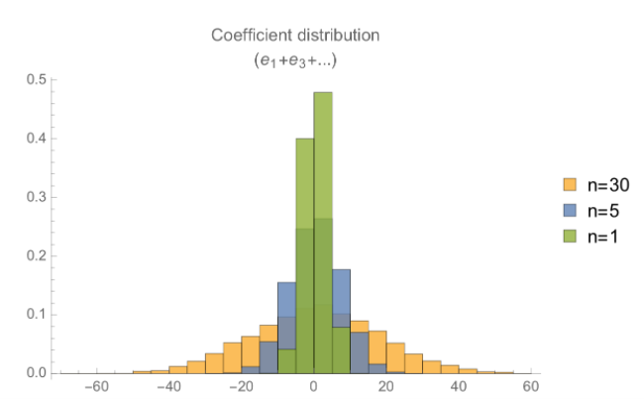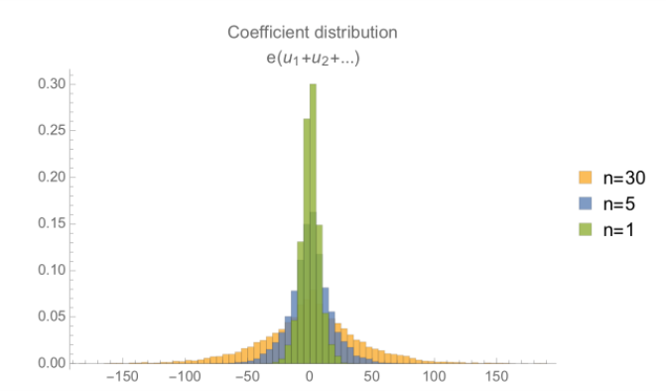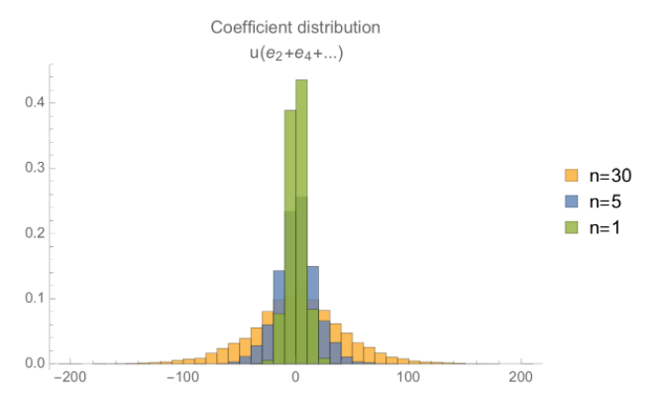## 同态乘法

$[CT_0+CT_1s^1]_q$

$[CT_0+CT_1s^1]_q \rightarrow \frac{q}{t}m+noise$

\begin{aligned} [a_0+a_1s^1]_q\rightarrow\frac{q}{t}m_1+n_1 \\ [b_0+b_1s^1]_q\rightarrow\frac{q}{t}m_2+n_2 \end{aligned}

$[a_0+a_1s^1]_q[b_0+b_1s^1]_q\rightarrow(\frac{q}{t}m_1+n_1)(\frac{q}{t}m_2+n_2)$

$[\lfloor \cfrac{t}{q}[CT_0*s^0+CT_1*s^1+CT_2*s^2]_q \rceil]_t$

\begin{aligned} a = [PK_0u_1+e_{11}+qm_1/t,PK_1u_1+e_{12}] \\ b = [PK_0u_2+e_{21}+qm_2/t,PK_1u_2+e_{22}] \end{aligned}

\begin{aligned} & CT_0*s^0+CT_1*s^1+CT_2*s^2= \\ & \frac{q}{t}m_1m_2+e_{22}m_1s+e_{12}m_2s+em_2u_1+em_1u_2+e_{21}m_1+e_{11}m_2 \\ & +\frac{t}{q}e^2u_1u_2+\frac{t}{q}e_{12}e_{22}s^2+\frac{t}{q}e_{22}esu_1+\frac{t}{q}e_{12}esu_2 \\ & +\frac{t}{q}e_{12}e_{21}s+\frac{t}{q}e_{11}e_{22}s+\frac{t}{q}e_{21}eu_1+\frac{t}{q}e_{11}eu_2+\frac{t}{q}e_{11}e_{21} \end{aligned}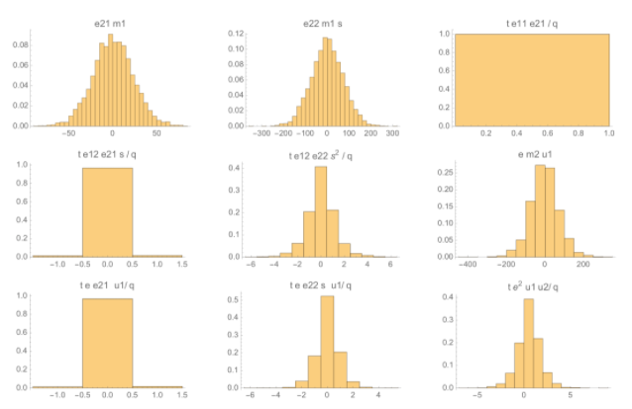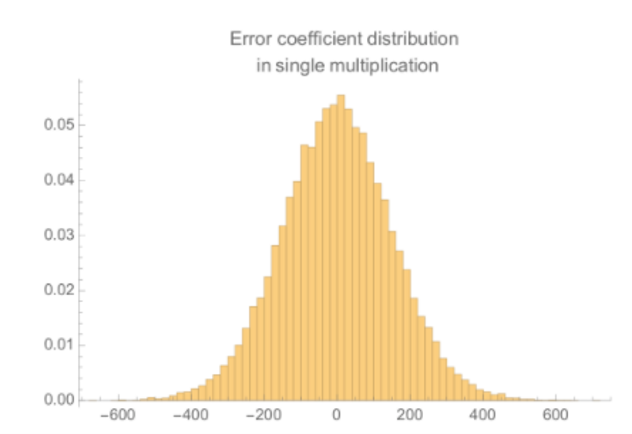## Relinearisation 和其他

Relinearisation 和这些“打包”的编码机制将在后续博文中进一步讨论。